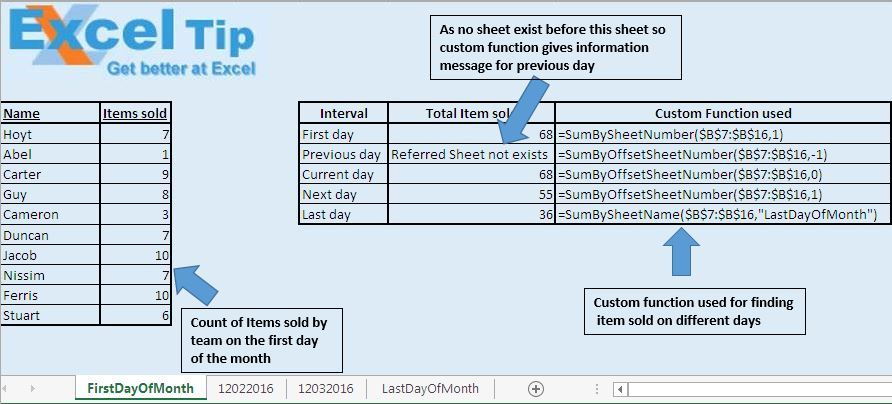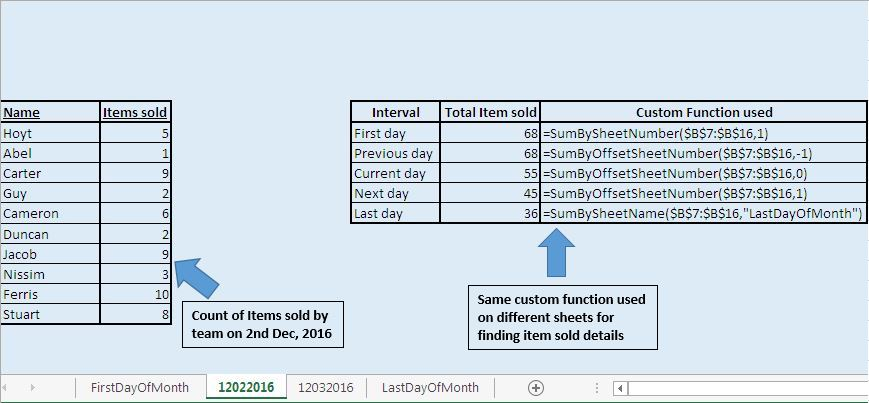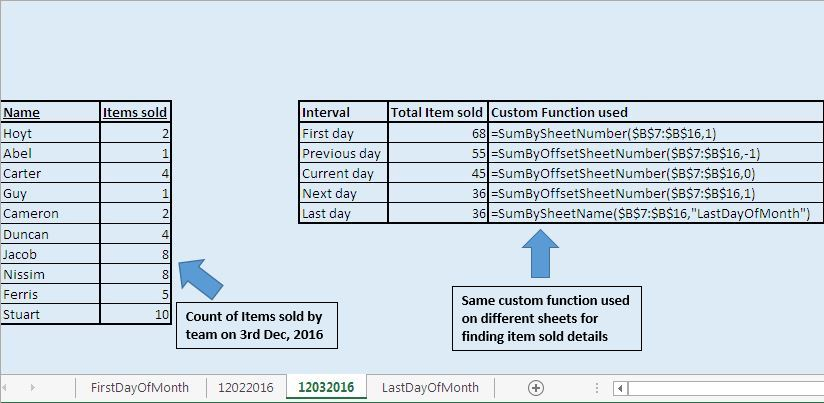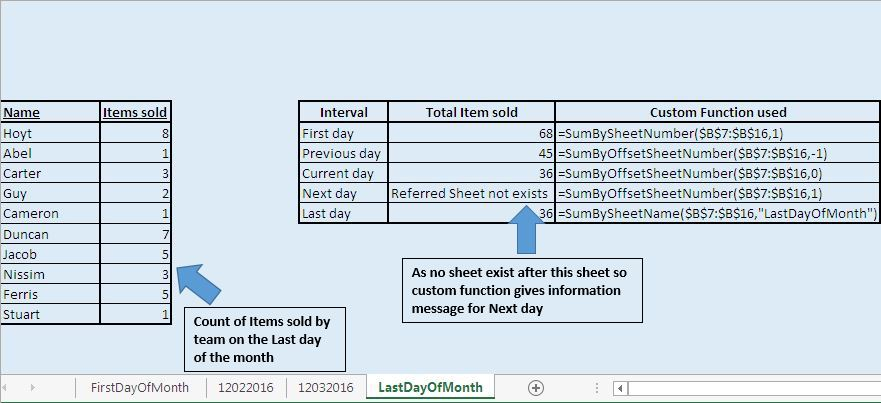# Add values from the previous or next worksheet using VBA in Microsoft Excel

In this article, we will create a custom function to calculate sum of values in the range of different sheets.

We will create three different custom functions which will calculate sum of values in the range of other sheets, based on sheet name, sheet number and sheet reference from the active sheet.

Raw data for this example consists of four sheets. Each sheet having details of number of items sold by each team member in the range B7 to B16 for a particular day. We have taken data for the 1st day, 2nd, 3rd and last day of the month.Logic explanation

In this example, we have created three custom functions that are: -

1. SumBySheetName

2. SumBySheetNumber

3. SumByOffsetSheetNumber

1. SumBySheetName: - This custom function takes the range and sheet name as input parameters. It returns the sum of values in the defined range of the defined sheet name.

1. SumBySheetNumber: - This custom function takes the range and sheet number as input parameters. It returns sum of the values in the defined range for the defined sheet number.

1. SumByOffsetSheetNumber: - This function takes the range and offset from the active sheet as input parameters. It returns sum of the values in the defined range of the sheet defined by the offset index.

Each sheet in the workbook show total items sold on the first day, previous day, current day, next day and last day.

If you have a close look at the custom function, you will find that all the four sheets use the same custom function with same parameters.

“SumBySheetNumber” custom function is used to calculate total items sold on the first day, as first sheet will always carry data of first day of the month.

“SumByOffsetSheetNumber” custom function is used to calculate total items sold on the previous, current and next day as sheets in the workbook are arranged in date sequence.

“SumBySheetName” custom function is used to calculate total items sold on the last day of the month, as sheet name of the last day will always be “LastDayOfMonth”.Code explanation

WorksheetFunction.Sum

WorksheetFunction object is used to access Excel sheet functions from Visual Basic. We have used SUM function of the Excel sheet to add the values in the range.

Above code is used to return the address of the specified range by “InputRange” range object.

```
Option Explicit

Function SumBySheetName(InputRange As Range, Optional SheetName As Variant)

'Declaring variable
Dim SheetIn As Worksheet

'Checking whether value is assigned to optional parameter
'IF optional parameter is missing then generate sum for range in the active sheet
If IsMissing(SheetName) Then
SumBySheetName = WorksheetFunction.Sum(InputRange)
Exit Function
End If

'Looping through sheets in the worksheets collection
For Each SheetIn In Worksheets
'Checking whether specified sheet exist in the worksheets collection
If SheetName = SheetIn.Name Then
'Calculating the sum for specified range of the specified sheet
Exit Function
End If
Next

SumBySheetName = "Specified Sheet doesn't exist in the workbook"

End Function

Function SumBySheetNumber(InputRange As Range, Optional SheetIndex As Integer = 0)

'Checking whether optional parameter is missing or sheet index is assigned zero value
If SheetIndex = 0 Then
SumBySheetNumber = WorksheetFunction.Sum(InputRange)
'Checking whether sheet index is greater than number of sheets in the workbook
ElseIf SheetIndex > Sheets.Count Then
SumBySheetNumber = "Sheet Index is greater than number of sheets in the workbook"
Else
End If

End Function

Function SumByOffsetSheetNumber(InputRange As Range, Optional SheetOffset As Integer = 0)

'Error Handling
On Error GoTo Last

'Calculating sum
SumByOffsetSheetNumber = WorksheetFunction.Sum(Worksheets(InputRange.Worksheet.Index + _

Exit Function

Last:
SumByOffsetSheetNumber = "Referred Sheet not exists"

End Function

```